# Tillson T3 Moving Average for Thinkorswim#### netarchitech

##### Well-known member
VIP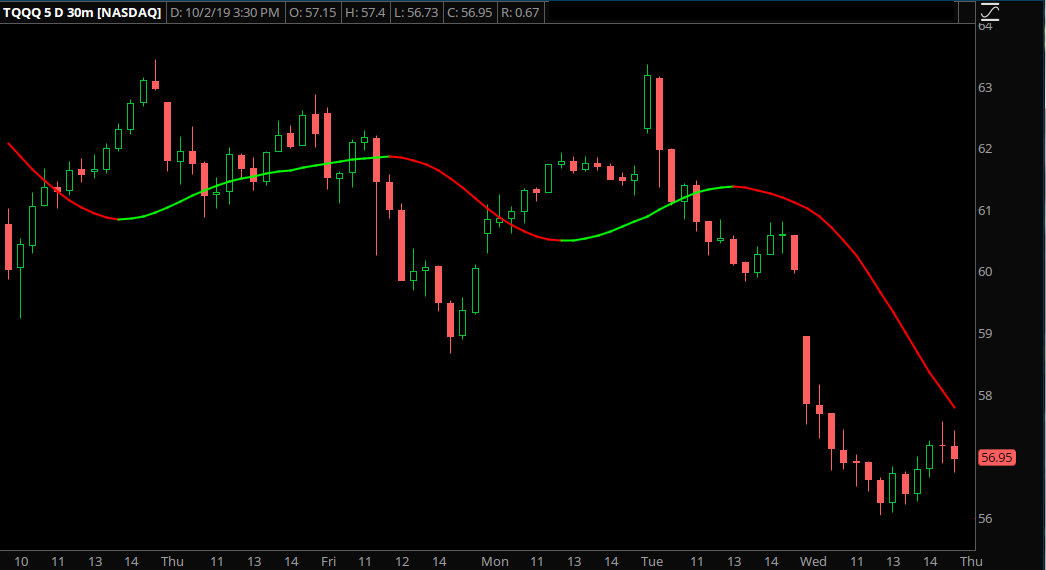Code:
``````# filename: _Tillson_T3_Moving_Average_
# source: https://futures.io/thinkorswim/34287-tilson-t3-moving-average.html#post460861
# created by: rmejia
# last update: 12/17/2014

#hint:<b>T3 Adaptive Smoothing Indicator</b>\nThis study was adopted from the Technical Analysis of Stocks and Commodities article "Smoothing Techniques for More Accurate Signals" by Tim Tillson, Jan 1998 (V16:1 pp33-37)
#hint: indicator: Defines the level of filtering to occur, default is 3
#hint: volumeFactor: Adjusts the amplitude of the feedback added back into the base filter

declare upper;

input indicator    = { T1, T2, default T3, T4, T5, T6 };
input price        = close;
input period       = 15;
input volumeFactor = 0.70;
input displace = 0;
input sign         = { default plus, minus };
input Label        = No;
input paintbars    = No;

script _gd {
input _price  = close;
input _period = 10;
input _v      = 0.70;
input _sign   = { default plus, minus };
def _ema      = ExpAverage( _price, _period );
plot _gd      = ( _ema * ( 1 + _v ) ) - ( ExpAverage( _ema, _period ) * _v );
}

def _t1 = _gd( price[-displace], period, volumeFactor, sign );
def _t2 = _gd( _t1,   period, volumeFactor, sign );
def _t3 = _gd( _t2,   period, volumeFactor, sign );
def _t4 = _gd( _t3,   period, volumeFactor, sign );
def _t5 = _gd( _t4,   period, volumeFactor, sign );
def _t6 = _gd( _t5,   period, volumeFactor, sign );

plot T3;
switch( indicator ) {
case T1:
T3 = _t1;
case T2:
T3 = _t2;
case T3:
T3 = _t3;
case T4:
T3 = _t4;
case T5:
T3 = _t5;
case T6:
T3 = _t6;
}

T3.AssignValueColor(if T3 > T3 then Color.GREEN else Color.RED);
T3.HideBubble();

AddLabel(Label, if T3 > T3 then "  A  " else "  A  ", if T3 > T3 then Color.GREEN else Color.RED);

assignPriceColor(if paintbars and T3 < T3 then color.DARK_RED else if paintbars and T3 > T3 then color.DARK_GREEN else color.CURRENT);``````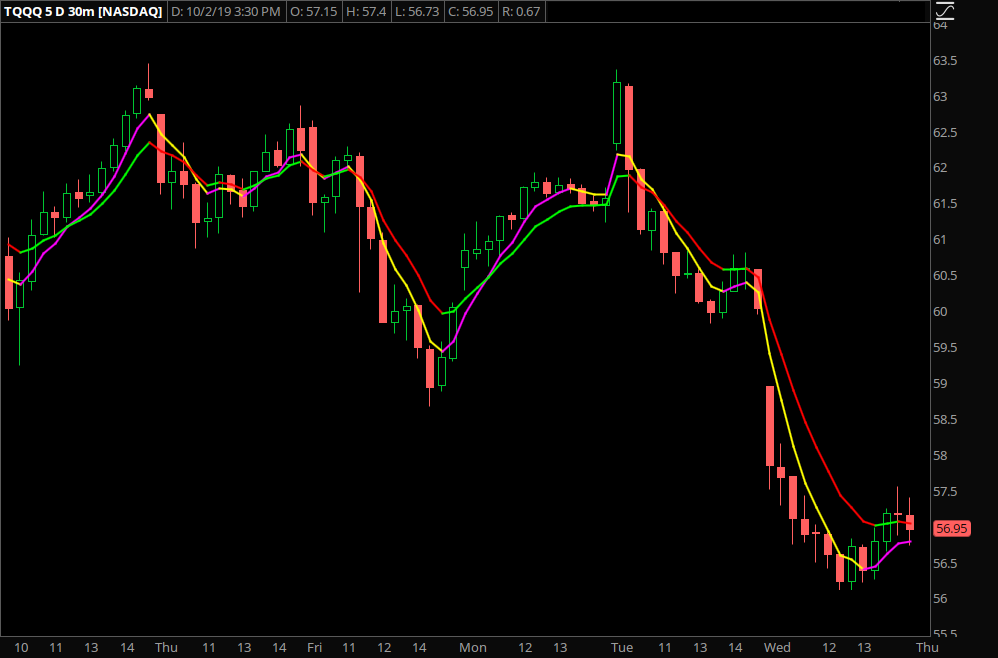Comparing 8 period EMA (Green/Red) with 8 period Tillson T3 MA with T1 setting (Magenta/Yellow)...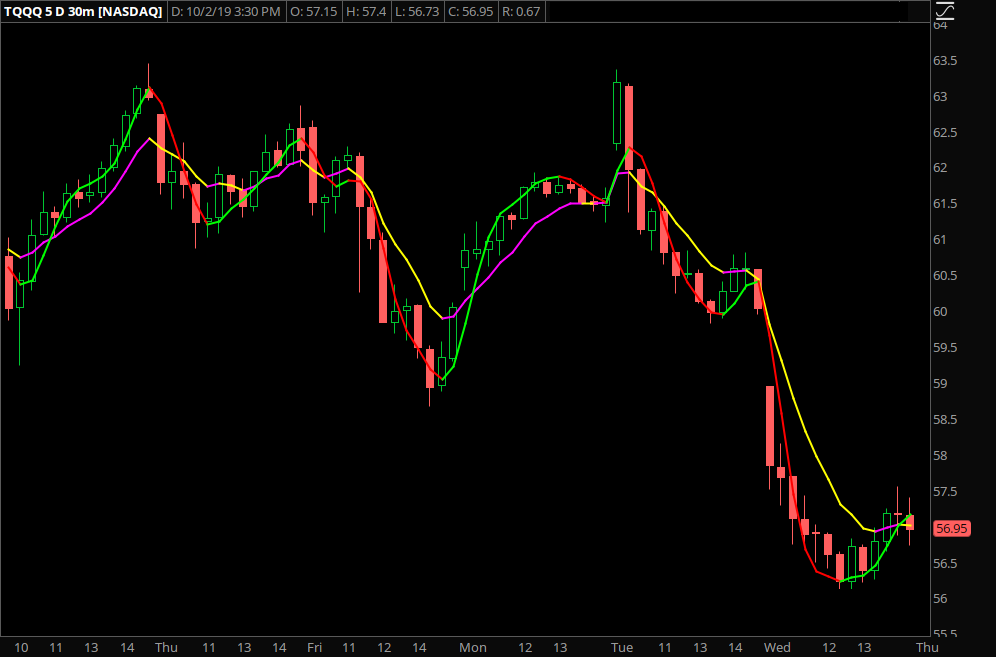Comparing 8 period Hull MA (Green/Red) with 8 period Tillson T3 MA with T1 setting (Magenta/Yellow)...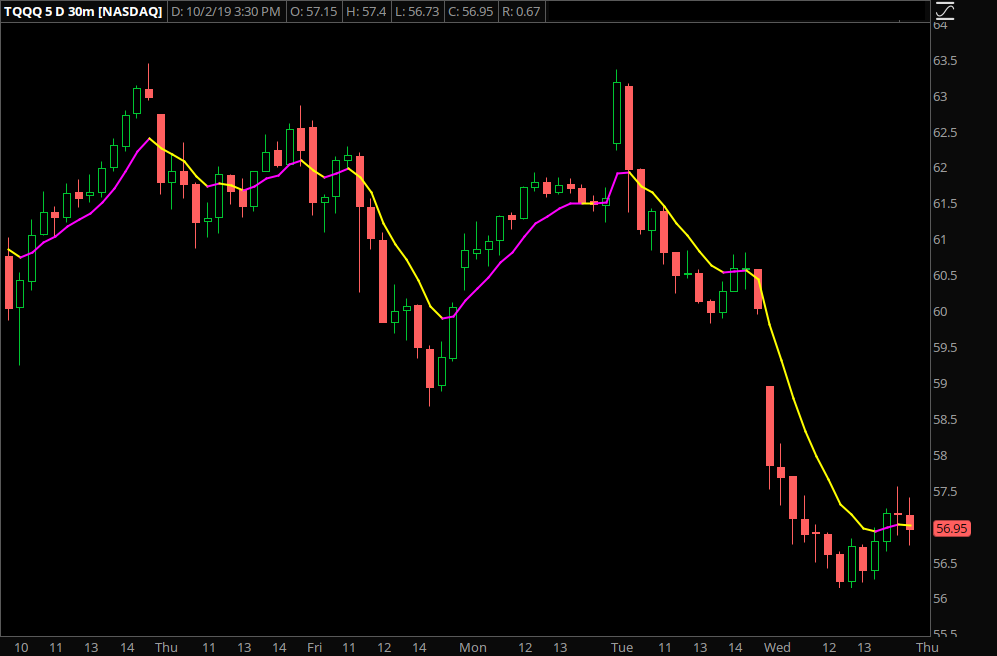The "Winner": 8 period Tillson T3 MA with T1 and 0.10 Volume Factor settings...

Last edited by a moderator:#### markos

##### Well-known member
VIP
Actually, I think the Hull is faster.Hull's turn is taken at polarity change, even if it "overshoots". Take a look at the bottom of that chart.
One day, I'll have to look for the monster comparo of ma's. Most are in the eye of the beholder.#### netarchitech

##### Well-known member
VIP
Actually, I think the Hull is faster.
@markos You are correct, sirI did the comparison to see which MA would keep you in a trend the longest. I guess I felt the Tillson T3 provided a little buffer between it and price. The Hull seems to plot directly on a sizable number of candles as it draws, which isn't helpful, IMO...

Most are in the eye of the beholder.
Very true...

As for the monster comparo, that would be interesting indeedT

##### Active member
2019 Donor
Could someone turn this into an MTF version?
Code:
``````# filename: Tillson_T3_Moving_Average
# source: https://futures.io/thinkorswim/34287-tilson-t3-moving-average.html#post460861
# created by: rmejia
# last update: 12/17/2014

#hint:<b>T3 Adaptive Smoothing Indicator</b>\nThis study was adopted from the Technical Analysis of Stocks and Commodities article "Smoothing Techniques for More Accurate Signals" by Tim Tillson, Jan 1998 (V16:1 pp33-37)
#hint: indicator: Defines the level of filtering to occur, default is 3
#hint: volumeFactor: Adjusts the amplitude of the feedback added back into the base filter

declare upper;

input indicator    = { T1, T2, default T3, T4, T5, T6 };
input price        = close;
input period       = 15;
input volumeFactor = 0.70;
input displace = 0;
input sign         = { default plus, minus };
input Label        = No;
input paintbars    = No;

script _gd {
input _price  = close;
input _period = 10;
input _v      = 0.70;
input _sign   = { default plus, minus };
def _ema      = ExpAverage( _price, _period );
plot _gd      = ( _ema * ( 1 + _v ) ) - ( ExpAverage( _ema, _period ) * _v );
}

def _t1 = _gd( price[-displace], period, volumeFactor, sign );
def _t2 = _gd( _t1,   period, volumeFactor, sign );
def _t3 = _gd( _t2,   period, volumeFactor, sign );
def _t4 = _gd( _t3,   period, volumeFactor, sign );
def _t5 = _gd( _t4,   period, volumeFactor, sign );
def _t6 = _gd( _t5,   period, volumeFactor, sign );

plot T3;
switch( indicator ) {
case T1:
T3 = _t1;
case T2:
T3 = _t2;
case T3:
T3 = _t3;
case T4:
T3 = _t4;
case T5:
T3 = _t5;
case T6:
T3 = _t6;
}

T3.AssignValueColor(if T3 > T3 then Color.GREEN else Color.RED);
T3.HideBubble();

AddLabel(Label, if T3 > T3 then "  A  " else "  A  ", if T3 > T3 then Color.GREEN else Color.RED);

assignPriceColor(if paintbars and T3 < T3 then color.DARK_RED else if paintbars and T3 > T3 then color.DARK_GREEN else color.CURRENT);``````
anyone around could kindly do this? Thanks

@markos is it possible to add a MTF to this ?

Last edited by a moderator:
H

#### HighBredCloud

##### Well-known member
VIP
^^^yeah this would be nice to have...for anyone with free time.

D

#### diazlaz

##### Well-known member
2019 Donor
VIP
Hi @Trading51, can you share some more information, how would you use T3 in MTF, what's the configuration and parameters you would use in the MTF?

•T

##### Active member
2019 Donor
@diazlaz think as it as a guide, if the 15 min is sloped red and the smaller time frame when it resets and flips back to align with the 15 min you have a small momentum trade, it makes it easier having the larger time frame visual verse having a bunch of charts open.

@BenTen you should have a small service added for a small fee for little suggests that need to be done, verse asking people for help, that's a good way to set up another fee-based scenario for this community, verse charging monthly give a few different options and subcontract the work out to different guys that can do it, this way they get a fee and so do you, juts a thought.

Last edited by a moderator:
•diazlaz
D

#### diazlaz

##### Well-known member
2019 Donor
VIP
Hi @Trading51, Here is an MTF version. please adjust your agg periods (1=A, 2=B), Label and color paints for the first Agg period included. please share your backtest and findings on how we might be able to trade thisI'm sure this can be further enhanced but wanted to refactor the gd script to make it MTF as an initial release. took me a bit of work and testing to make sure it was correct.Ruby:
``````#Tillson T3 Moving Average MTF Edition for ThinkOrSwim
#@diazlaz 2020.03.31 Version 1.0
#
# filename: Tillson_T3_Moving_Average
# source: https://futures.io/thinkorswim/34287-tilson-t3-moving-average.html#post460861
# created by: rmejia
# last update: 12/17/2014
# MTF Version @diazlaz 2020.03.31 Version 1.0
#hint:<b>T3 Adaptive Smoothing Indicator</b>\nThis study was adopted from the Technical Analysis of Stocks and Commodities article "Smoothing Techniques for More Accurate Signals" by Tim Tillson, Jan 1998 (V16:1 pp33-37)
#hint: indicator: Defines the level of filtering to occur, default is 3
#hint: volumeFactor: Adjusts the amplitude of the feedback added back into the base filter
input indicator    = { T1, T2, default T3, T4, T5, T6 };
input price = close;
input period = 15;
input volumeFactor = 0.70;
input displace = 0;
input sign = { plus, default minus };
input Label = Yes;
input paintbars    = Yes; #paints Primary aggregation1
input aggregation1 = AggregationPeriod.MIN;
input aggregation2 = AggregationPeriod.FIVE_MIN;
#aggregation1
#T1
def t1_price_01 = close(period = aggregation1);
def t1_ema_01 = ExpAverage( t1_price_01, period );
def t1_gd_01 = ( t1_ema_01 * ( 1 + volumeFactor ) ) - ( ExpAverage( t1_ema_01, period ) * volumeFactor );
#T2
def t2_price_01 = t1_gd_01;
def t2_ema_01 = ExpAverage( t2_price_01, period );
def t2_gd_01 = ( t2_ema_01 * ( 1 + volumeFactor ) ) - ( ExpAverage( t2_ema_01, period ) * volumeFactor );
#T3
def t3_price_01 = t2_gd_01;
def t3_ema_01 = ExpAverage( t3_price_01, period );
def t3_gd_01 = ( t3_ema_01 * ( 1 + volumeFactor ) ) - ( ExpAverage( t3_ema_01, period ) * volumeFactor );
#T4
def T4_price_01 = t3_gd_01;
def T4_ema_01 = ExpAverage( T4_price_01, period );
def T4_gd_01 = ( T4_ema_01 * ( 1 + volumeFactor ) ) - ( ExpAverage( T4_ema_01, period ) * volumeFactor );
#T5
def T5_price_01 = T4_gd_01;
def T5_ema_01 = ExpAverage( T5_price_01, period );
def T5_gd_01 = ( T5_ema_01 * ( 1 + volumeFactor ) ) - ( ExpAverage( T5_ema_01, period ) * volumeFactor );
#T6
def T6_price_01 = T5_gd_01;
def T6_ema_01 = ExpAverage( T6_price_01, period );
def T6_gd_01 = ( T6_ema_01 * ( 1 + volumeFactor ) ) - ( ExpAverage( T6_ema_01, period ) * volumeFactor );
plot T3_01;
switch( indicator ) {
case T1:
T3_01 = t1_gd_01;
case T2:
T3_01 = t2_gd_01;
case T3:
T3_01 = t3_gd_01;
case T4:
T3_01 = t4_gd_01;
case T5:
T3_01 = t5_gd_01;
case T6:
T3_01 = t6_gd_01;
}
T3_01.AssignValueColor(if T3_01 > T3_01 then Color.GREEN else Color.RED);
T3_01.HideBubble();
AddLabel(Label, if T3_01 > T3_01 then " A " else " A ", if T3_01 > T3_01 then Color.GREEN else Color.RED);
assignPriceColor(if paintbars and T3_01 < T3_01 then color.DARK_RED else if paintbars and T3_01 > T3_01 then color.DARK_GREEN else color.CURRENT);
#aggregation2
#T1
def t1_price_02 = close(period = aggregation2);
def t1_ema_02 = ExpAverage( t1_price_02, period );
def t1_gd_02 = ( t1_ema_02 * ( 1 + volumeFactor ) ) - ( ExpAverage( t1_ema_02, period ) * volumeFactor );
#T2
def t2_price_02 = t1_gd_02;
def t2_ema_02 = ExpAverage( t2_price_02, period );
def t2_gd_02 = ( t2_ema_02 * ( 1 + volumeFactor ) ) - ( ExpAverage( t2_ema_02, period ) * volumeFactor );
#T3
def t3_price_02 = t2_gd_02;
def t3_ema_02 = ExpAverage( t3_price_02, period );
def t3_gd_02 = ( t3_ema_02 * ( 1 + volumeFactor ) ) - ( ExpAverage( t3_ema_02, period ) * volumeFactor );
#T4
def T4_price_02 = t3_gd_02;
def T4_ema_02 = ExpAverage( T4_price_02, period );
def T4_gd_02 = ( T4_ema_02 * ( 1 + volumeFactor ) ) - ( ExpAverage( T4_ema_02, period ) * volumeFactor );
#T5
def T5_price_02 = T4_gd_02;
def T5_ema_02 = ExpAverage( T5_price_02, period );
def T5_gd_02 = ( T5_ema_02 * ( 1 + volumeFactor ) ) - ( ExpAverage( T5_ema_02, period ) * volumeFactor );
#T6
def T6_price_02 = T5_gd_02;
def T6_ema_02 = ExpAverage( T6_price_02, period );
def T6_gd_02 = ( T6_ema_02 * ( 1 + volumeFactor ) ) - ( ExpAverage( T6_ema_02, period ) * volumeFactor );
plot T3_02;
switch( indicator ) {
case T1:
T3_02 = t1_gd_02;
case T2:
T3_02 = t2_gd_02;
case T3:
T3_02 = t3_gd_02;
case T4:
T3_02 = t4_gd_02;
case T5:
T3_02 = t5_gd_02;
case T6:
T3_02 = t6_gd_02;
}
T3_02.AssignValueColor(if T3_02 > T3_02 then Color.GREEN else Color.RED);
T3_02.setLineWeight(2);
T3_02.HideBubble();
AddLabel(Label, if T3_02 > T3_02 then "  B  " else "  B  ", if T3_02 > T3_02 then Color.GREEN else Color.RED);

#End of #Tillson T3 Moving Average MTF Edition for ThinkOrSwim``````

T

##### Active member
2019 Donor
@diazlaz Hey! Thanks for taking the time and helping out, will do!! Cheers mate be safe out there!.

•HighBredCloud and mashume#### horserider

##### Well-known member
VIP
Now how can I make \$5000. Darn it diazlaz.•Charlielee
T

##### Active member
2019 Donor
@diazlaz Do you trade futures or stocks

Is there a way to adjust the upward slope to be solid green just like when it slopes down its solid red?

Last edited by a moderator:#### horserider

##### Well-known member
VIP
You would need to change the assignvaluecolor. Just making this up and not testing it but maybe;
T3_02.AssignValueColor(if T3_02 > T3_02 and T3_02 = T3_02 then Color.GREEN else if T3_02 < T3_02 and T3_02 = T3_02 then Color.RED else Color.Gray);

•T

##### Active member
2019 Donor
You would need to change the assignvaluecolor. Just making this up and not testing it but maybe;
T3_02.AssignValueColor(if T3_02 > T3_02 and T3_02 = T3_02 then Color.GREEN else if T3_02 < T3_02 and T3_02 = T3_02 then Color.RED else Color.Gray);
Got an error#### horserider

##### Well-known member
VIP
Oh well that is what I get for just making things up. Sorry.

Just do what it says ,,, put in == for = and see if it works

D

#### diazlaz

##### Well-known member
2019 Donor
VIP
Updated as per request.Ruby:
``````#Tillson T3 Moving Average MTF Edition for ThinkOrSwim
#@diazlaz 2020.03.31 Version 1.1
#
# filename: Tillson_T3_Moving_Average
# source: https://futures.io/thinkorswim/34287-tilson-t3-moving-average.html#post460861
# created by: rmejia
# last update: 12/17/2014
# MTF Version @diazlaz 2020.03.31 Version 1.0
# Version 1.1 @diazlaz 2020.03.31 Version 1.1 - Updated Line Plots to plot both states at lower timeframe.
#
#hint:<b>T3 Adaptive Smoothing Indicator</b>\nThis study was adopted from the Technical Analysis of Stocks and Commodities article "Smoothing Techniques for More Accurate Signals" by Tim Tillson, Jan 1998 (V16:1 pp33-37)
#hint: indicator: Defines the level of filtering to occur, default is 3
#hint: volumeFactor: Adjusts the amplitude of the feedback added back into the base filter

input indicator    = { T1, T2, default T3, T4, T5, T6 };
input price = close;
input period = 15;
input volumeFactor = 0.70;
input displace = 0;
input sign = { plus, default minus };
input Label = Yes;
input paintbars    = Yes; #paints Primary aggregation1
input aggregation1 = AggregationPeriod.MIN;
input aggregation2 = AggregationPeriod.FIVE_MIN;
#aggregation1
#T1
def t1_price_01 = close(period = aggregation1);
def t1_ema_01 = ExpAverage( t1_price_01, period );
def t1_gd_01 = ( t1_ema_01 * ( 1 + volumeFactor ) ) - ( ExpAverage( t1_ema_01, period ) * volumeFactor );
#T2
def t2_price_01 = t1_gd_01;
def t2_ema_01 = ExpAverage( t2_price_01, period );
def t2_gd_01 = ( t2_ema_01 * ( 1 + volumeFactor ) ) - ( ExpAverage( t2_ema_01, period ) * volumeFactor );
#T3
def t3_price_01 = t2_gd_01;
def t3_ema_01 = ExpAverage( t3_price_01, period );
def t3_gd_01 = ( t3_ema_01 * ( 1 + volumeFactor ) ) - ( ExpAverage( t3_ema_01, period ) * volumeFactor );
#T4
def T4_price_01 = t3_gd_01;
def T4_ema_01 = ExpAverage( T4_price_01, period );
def T4_gd_01 = ( T4_ema_01 * ( 1 + volumeFactor ) ) - ( ExpAverage( T4_ema_01, period ) * volumeFactor );
#T5
def T5_price_01 = T4_gd_01;
def T5_ema_01 = ExpAverage( T5_price_01, period );
def T5_gd_01 = ( T5_ema_01 * ( 1 + volumeFactor ) ) - ( ExpAverage( T5_ema_01, period ) * volumeFactor );
#T6
def T6_price_01 = T5_gd_01;
def T6_ema_01 = ExpAverage( T6_price_01, period );
def T6_gd_01 = ( T6_ema_01 * ( 1 + volumeFactor ) ) - ( ExpAverage( T6_ema_01, period ) * volumeFactor );
plot T3_01;
switch( indicator ) {
case T1:
T3_01 = t1_gd_01;
case T2:
T3_01 = t2_gd_01;
case T3:
T3_01 = t3_gd_01;
case T4:
T3_01 = t4_gd_01;
case T5:
T3_01 = t5_gd_01;
case T6:
T3_01 = t6_gd_01;
}
T3_01.AssignValueColor(if T3_01 > T3_01 then Color.GREEN else Color.RED);
T3_01.HideBubble();
AddLabel(Label, if T3_01 > T3_01 then " A " else " A ", if T3_01 > T3_01 then Color.GREEN else Color.RED);
assignPriceColor(if paintbars and T3_01 < T3_01 then color.DARK_RED else if paintbars and T3_01 > T3_01 then color.DARK_GREEN else color.CURRENT);
#aggregation2
#T1
def t1_price_02 = close(period = aggregation2);
def t1_ema_02 = ExpAverage( t1_price_02, period );
def t1_gd_02 = ( t1_ema_02 * ( 1 + volumeFactor ) ) - ( ExpAverage( t1_ema_02, period ) * volumeFactor );
#T2
def t2_price_02 = t1_gd_02;
def t2_ema_02 = ExpAverage( t2_price_02, period );
def t2_gd_02 = ( t2_ema_02 * ( 1 + volumeFactor ) ) - ( ExpAverage( t2_ema_02, period ) * volumeFactor );
#T3
def t3_price_02 = t2_gd_02;
def t3_ema_02 = ExpAverage( t3_price_02, period );
def t3_gd_02 = ( t3_ema_02 * ( 1 + volumeFactor ) ) - ( ExpAverage( t3_ema_02, period ) * volumeFactor );
#T4
def T4_price_02 = t3_gd_02;
def T4_ema_02 = ExpAverage( T4_price_02, period );
def T4_gd_02 = ( T4_ema_02 * ( 1 + volumeFactor ) ) - ( ExpAverage( T4_ema_02, period ) * volumeFactor );
#T5
def T5_price_02 = T4_gd_02;
def T5_ema_02 = ExpAverage( T5_price_02, period );
def T5_gd_02 = ( T5_ema_02 * ( 1 + volumeFactor ) ) - ( ExpAverage( T5_ema_02, period ) * volumeFactor );
#T6
def T6_price_02 = T5_gd_02;
def T6_ema_02 = ExpAverage( T6_price_02, period );
def T6_gd_02 = ( T6_ema_02 * ( 1 + volumeFactor ) ) - ( ExpAverage( T6_ema_02, period ) * volumeFactor );
plot T3_02;
switch( indicator ) {
case T1:
T3_02 = t1_gd_02;
case T2:
T3_02 = t2_gd_02;
case T3:
T3_02 = t3_gd_02;
case T4:
T3_02 = t4_gd_02;
case T5:
T3_02 = t5_gd_02;
case T6:
T3_02 = t6_gd_02;
}

def sState1 = if T3_01 > T3_01 then 100 else -100;
def sState2 = if T3_02 > T3_02 then 100 else -100;
T3_02.AssignValueColor(if sState2 == 100 then Color.GREEN else Color.RED);
T3_02.setLineWeight(2);
T3_02.HideBubble();
AddLabel(Label, if T3_02 > T3_02 then "  B  " else "  B  ", if T3_02 > T3_02 then Color.GREEN else Color.RED);

#End of #Tillson T3 Moving Average MTF Edition for ThinkOrSwim``````

T

##### Active member
2019 Donor
@diazlaz ThankYOU!!!!

•diazlaz
D

#### diazlaz

##### Well-known member
2019 Donor
VIP
@diazlaz ThankYOU!!!!
happy trading!! - let us know what you find! all the best.

•T

##### Active member
2019 Donor
happy trading!! - let us know what you find! all the best.
@diazlaz I'm using it on the 15 minute and 3 minutes and I'm using the slope for a directional guide it catches decent size swings, take a look at any futures contract you will see what I mean, let me know what you think.

•diazlaz
T

##### Active member
2019 Donor
happy trading!! - let us know what you find! all the best.
@diazlaz I have another code that would work really good with this if you could add a MTF to it when you get a chance let me know.

•brmeehan
D

#### diazlaz

##### Well-known member
2019 Donor
VIP
@diazlaz I have another code that would work really good with this if you could add a MTF to it when you get a chance let me know.
post it here or create a new post if it's not relevant to T3 and let's see what we can do.

•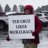Thinkscript for Moving Average from different Index/Indicator Questions 2